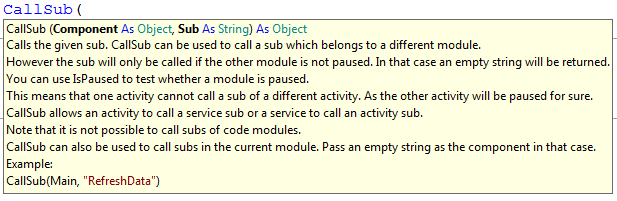# Android QuestionCallSub warning #25

#### mackrackit

##### Member
Longtime User
Hi,
My code works but I am also receiving a warning.
First I have a variable,
B4X:
``````Sub Globals
Dim ZLetter As String
End Sub``````
The warning is on the "CallSub" line.
B4X:
``````Sub GPS_LocationChanged (Location1 As Location)
CallSub(ZoneLetter,"ZLetter")
lblZone.Text = "Zone = " & Zone & ZLetter
End Sub``````
And this is the sub called
B4X:
``````Sub ZoneLetter
If((84 >= Latx) AND (Latx >= 72)) Then
ZLetter = "X"
Else If((72 > Latx) AND (Latx >= 64)) Then
ZLetter = "W"
Else If((64 > Latx) AND (Latx >= 56)) Then
ZLetter = "V"
Else If((56 > Latx) AND (Latx >= 48)) Then
ZLetter = "U"
Else If((48 > Latx) AND (Latx >= 40)) Then
ZLetter = "T"
Else If((40 > Latx) AND (Latx >= 32)) Then
ZLetter = "S"
Else If((32 > Latx) AND (Latx >= 24)) Then
ZLetter = "R"
Else If((24 > Latx) AND (Latx >= 16)) Then
ZLetter = "Q"
Else If((16 > Latx) AND (Latx >= 8)) Then
ZLetter = "P"
Else If(( 8 > Latx) AND (Latx >= 0)) Then
ZLetter = "N"
Else If(( 0 > Latx) AND (Latx >= -8)) Then
ZLetter = "M"
Else If((-8> Latx) AND (Latx >= -16)) Then
ZLetter = "L"
Else If((-16 > Latx) AND (Latx >= -24)) Then
ZLetter = "K"
Else If((-24 > Latx) AND (Latx >= -32)) Then
ZLetter = "J"
Else If((-32 > Latx) AND (Latx >= -40)) Then
ZLetter = "H"
Else If((-40 > Latx) AND (Latx >= -48)) Then
ZLetter = "G"
Else If((-48 > Latx) AND (Latx >= -56)) Then
ZLetter = "F"
Else If((-56 > Latx) AND (Latx >= -64)) Then
ZLetter = "E"
Else If((-64 > Latx) AND (Latx >= -72)) Then
ZLetter = "D"
Else If((-72 > Latx) AND (Latx >= -80)) Then
ZLetter = "C"
Else
ZLetter = "Z"
End If

End Sub``````

#### LucaMs

##### Expert
Longtime User
You should read the tip (intellisense) for the CallSub statement:If your function is in the same Activity you should use:
CallSub("", "ZoneLetter").

Better yet, do not use the global variable, but pass it to the function:
CallSub2("", "ZoneLetter", ZLetter)

#### mackrackit

##### Member
Longtime User
Thank you!!!

I was reading the tip wrong, it is amazing that it worked at all.